×#### Thank you for registering.

One of our academic counsellors will contact you within 1 working day.

Click to Chat

1800-1023-196

+91-120-4616500

CART 0

• 0

MY CART (5)

Use Coupon: CART20 and get 20% off on all online Study Material

ITEM
DETAILS
MRP
DISCOUNT
FINAL PRICE
Total Price: Rs.

There are no items in this cart.
Continue ShoppingDefinite Integral

Table of Content

The topics covered under it are listed below

What do you mean by a definite integral?

How is indefinite integral different from definite integral?

Some of the important properties of definite integrals are listed below

Some Important Expansions

Related ResourcesDefinite integral is generally considered to be a tough topic by students. It must be studied after one is thorough with the concepts of indefinite integrals. The topic is flooded with formulae related to change of limits etc. and hence demands consistent practice. It is an important component of the IIT JEE Mathematics syllabus and so students cannot dare to skip this topic. It not only requires learning of all important formulae but also requires a good speed with thorough knowledge of integration.

Many students grasp integration but make silly errors when completing the two part calculation. Simple peer assessment could help students to think about setting each step of their work minimizing these small but significant slips. This work could be made more engaging by inviting students to mix and match integration problems with graphical representations. Broadly speaking, this section also forms the root of all scientific organizations and studies.

The topics covered under it are listed below

Geometrical Interpretation of Definite Integral

Fundamental Theorems of Calculus

Properties of Definite Integration

Differentiation Under Integral Sign

Definite Integral as Limit of Sum

Area as Definite Integral

Working Rule for Finding Area

Objective Problems of Definite Problems

Solved Examples of Area Definite Integral

Area Bounded by Parabola Straight Line Circle

Solved Examples of Definite Integral

These heads have been discussed in detail in the coming sections. Here we shall discuss them in brief:

What do you mean by a definite integral?

A quantity of the form

is called the definite integral of f(x) between the limits ‘a’ and ‘b’, where d/dx (F(x)) = f(x).

How is indefinite integral different from definite integral?

Indefinite integral lays down the foundation for definite integral. Both the concepts are synonymous and interrelated. As the name suggests, while indefinite integral refers to the evaluation of indefinite area, in definite integrationthe area is to be calculated within specific limits. The figure given below illustrates clearly the difference between definite and indefinite integration: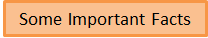Some of the important properties of definite integrals are listed below

The value of the integral is unaffected by the variable of integration as long as the limits of integration remain the same.

?

If the limits of integration are interchanged, the value of integration remains same but the sign changes.

If the value of the integral is zero, then the equation f(x) = 0 has at least one root in (a, b) provided f is continuous in (a, b).

The converse of the above result is not true.

where c is a point lying inside or outside the interval [a, b], provided the function ‘f’ is piecewise continuous in (a, b).

where ‘a’ is the period of the function i.e. f(a + x) = f(x).

where f(x) is periodic with period ‘a’.where K = π/2, if both m and n are even

= 1 otherwise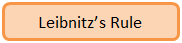If g is continuous on [a, b] and f1 (x) and f2 (x) are differentiable functions whose values lie in [a, b], then d/dx ∫ f2(x) f1(x) g(t) dt = g (f2(x)) f2'(x) – g (f1(x)) f1'(x)Express the given series in the form ∑ 1/n f (r/n)

Then the limit is its sum when n→∞, i.e. lim n→∞ h ∑1/n f(r/n)

Replace r/n by x and 1/n by dx and lim n→∞ ∑ by the sign of ∫.

The lower and the upper limit of integration are the limiting values of r/n for the first and the last term of r respectively.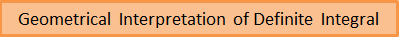The definite integral can be interpreted to represent the area under the graph. This follows from the definition itself that the definite integral is a sum of the product of the lengths of intervals and the "height" of the function being integrated in that interval including the formula for the area of the rectangle. The figure given below clearly illustrates that as we find the value of the definite integral a to b, we are actually finding the area of the shaded portion.Some Important Expansions

1-1/2 + 1/3 – 1/4 + 1/5 – …..  ∞  = ln 2

1/12 + 1/22 + 1/32 + 1/42 + …. ∞ = π2/6

1/12 - 1/22 + 1/32 - 1/42 + …. ∞ = π2/12

1/12 + 1/32 + 1/52 + 1/72 + …. ∞ = π2/8

1/22 + 1/42 + 1/62 + 1/82 + …. ∞ = π2/24Solution:

Given that F(x) = ∫ f(t) dt, where integral runs from 0 to x.

By Leibnitz rule, we have

F’(x) = f(x)

But F(x2) = x2 (1+x) = x2 + x3

Hence, this gives F(x) = x + x3/2

So, F’(x) = 1+ 3/2 x1/2

Hence, f(x) = F’(x) = 1+ 3/2 x1/2

Hence, f(4) = 1+ 3/2 . (4)1/2

This gives f(4) = 1+ 3/2 . 2 = 4

Hence, option (3) is correct.

________________________________________________________________________________Solution:

F(x) = ∫√2-t2 dt, where the integral runs from 1 to x

Hence, f’(x) = √2-x2

Another condition that is given in the question is that x2 – f’(x) = 0

Therefore, x2 = √2-x2

Hence, x4 = 2-x2

So, x4 + x2 – 2 = 0

This gives the value of x as ±1.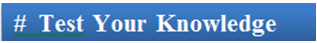Q1: In Leibnitz rule,

(a) the function f is continuous on [a, b] and the functions g1 and g2 are also continuous.

(b) the function f is discontinuous on [a, b] and the functions g1 and g2 are differentiable in [a, b].

(c) the function f is continuous on [a, b] and the functions g1 and g2 are discontinuous.

(d) the function f is continuous on [a, b] and the functions g1 and g2 are differentiable in [a, b].

Q2. If the upper and the lower limits are interchanged, the value of the integral

(a) remains same

(b) may or may not change

(c) value is same but the sign changes

(d) None of these

Q3. ?

(a) always holds good

(b) true provided f is piecewise continuous

(c) true if f is differentiable

(d) never true

Q4.

(a) f(2a-x) = -f(x)

(b) f(2a-x) = f(x)

(c) f(2a-x) = 0

(d) f(2a-x) = f(a+x)

Q5. Once definite integral is computed, the final result

(a) is a definite value without a constant (may be finite or infinite).

(b) may contain a constant

(c) always contains a constant

(d) contains a finite value along with a constant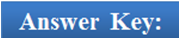Q1(d), Q2( c), Q3(b), Q4(a), Q5(a).

Related Resources

For getting an idea of the type of questions asked, refer the previous year papers.

You might like to refer Indefinite Integral.

To read more, Buy study materials of Definite integral comprising study notes, revision notes, video lectures, previous year solved questions etc. Also browse for more study materials on Mathematics here.### Course Features

• 731 Video Lectures
• Revision Notes
• Previous Year Papers
• Mind Map
• Study Planner
• NCERT Solutions
• Discussion Forum
• Test paper with Video Solution